# VBA VLOOKUP or XLOOKUP in Another Sheet or Workbook

This article will demonstrate how to use the VLOOKUP and XLOOPUP functions to lookup values in other sheets or workbooks in VBA.

The VLOOKUP and XLOOKUP functions in Excel are useful functions that allow us to search for a matching value in a range and return a corresponding value from another column. These functions can be used directly in a formula in Excel, or can be using in VBA Coding with the WorksheetFunction method. We can use these function to look up values that are contained in a different sheet from where we want the result to be produced, or even in a different workbook.

## VLOOKUP and XLOOKUP from Another Sheet

### VLOOKUP from Another Sheet

To use the VLOOKUP in a different sheet from the lookup data, we can type the following code:

``````Sub LookupPrice()
ActiveCell = Application.WorksheetFunction.VLookup(Range("B3"), Sheets("ProductList").Range("B2:C6"), 2, False)
End Sub``````

OR

``````Sub LookupPrice()
ActiveCell = Application.VLookup(Range("B3"), Sheets("ProductList").Range("B2:C6"), 2, False)
End Sub``````

Resulting in the following: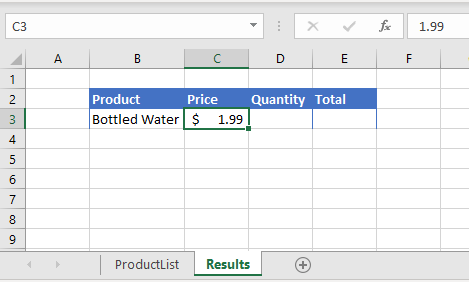We could also, for ease of use, use variables in our code.

``````Sub LookupPrice()
Dim ws As Worksheet
Dim rng As Range
Dim strProduct As String
strProduct = Range("B3")
Set ws = Sheets(1)
Set rng = ws.Range("B2:C6")
ActiveCell = Application.WorksheetFunction.VLookup(strProduct, rng, 2, False)
End Sub``````

This would return the same result.

If we are looking up a value that does not exist, we might want to use the Application.VLookup function to return #N/A to the cell rather than the Application.WorksheetFunction.VLookup function which would result in a VBA error.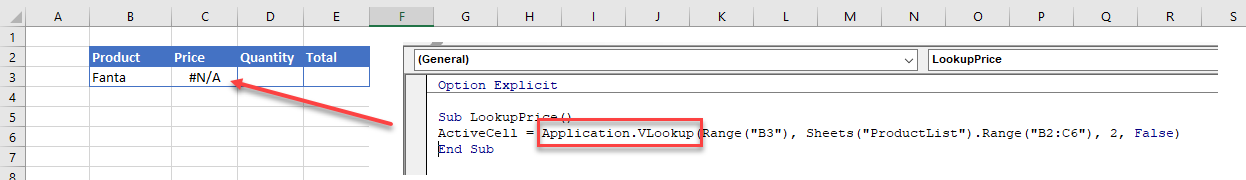If we wish to return the formula rather than the value to the target cell, then this is the code we would need to enter.

``````Sub LookupPrice()
ActiveCell = "=VLOOKUP(RC[-1],ProductList!RC[-1]:RC,2,FALSE)"
End Sub``````

Where the formula is using the R1C1 (rows and columns) syntax instead of the A1 (range) syntax.

This would result in: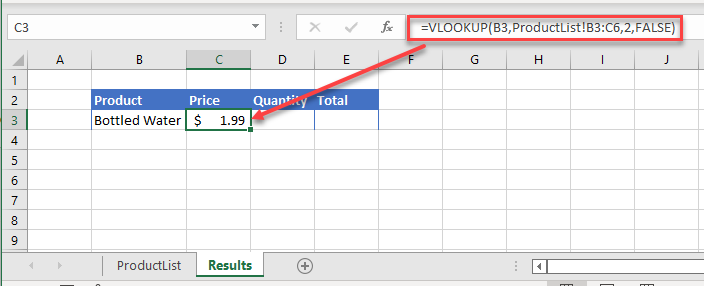### XLOOKUP from Another Sheet

Using the XLOOKUP to look up data in a different sheet is much the same. Remember that the XLOOKUP looks at multiple ranges rather than as a specific column to find the value.

``````Sub LookupPrice()
ActiveCell = Application.WorksheetFunction.XLookup(Range("B3"), Sheets("ProductList").Range("B2:B6"), Sheets("ProductList").Range("C2:C6"))
End Sub``````

or with variables:

``````Sub LookupPrice()
Dim ws As Worksheet
Dim strProduct As String
Dim rngProduct As Range
Dim rngPrice As Range
strProduct = Range("B3")
Set ws = Sheets("ProductList")
Set rngProduct = ws.Range("B2:B6")
Set rngPrice = ws.Range("C2:C6")
ActiveCell = Application.WorksheetFunction.XLookup(strProduct, rngProduct, rngPrice)
End Sub``````

The result of either of these examples would then be: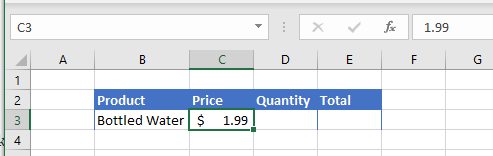To return a formula using XLOOKUP, we would once again need to use the row/column (R1C1) syntax rather than the Range (A1) syntax.

``````Sub EnterFormula
ActiveCell.FormulaR1C1 = "=XLOOKUP(RC[-1],ProductList!RC[-1]:RC[-1],ProductList!RC:RC)"
End Sub``````

The result of which would be: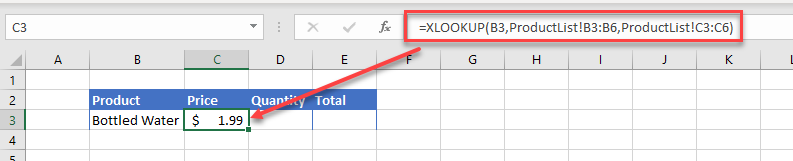One of the major advantages of using XLOOKUP vs VLOOKUP is the ability to lookup a range of columns, and return the value in each column.

Lets look at the following example: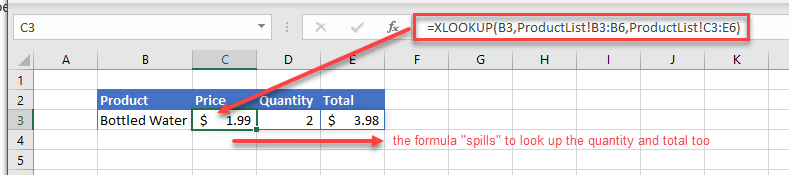We have created the formula in cell C3 where it is looking up the values in the range C2:E6.  Due to the fact that these are multiple columns, it will automatically populate columns D and E with he matching results found.  The formula SPILLS over into columns D and E without us haing to use CTRL+SHIFT for an array formula – this ability for the formula to SPILL across is one of the new additions for Excel 365.

To replicate this with VBA code, we can type the following in our macro:

``````Sub LookupPrice()
ActiveCell.Formula2R1C1 = "=XLOOKUP(RC[-1],ProductList!RC[-1]:RC[-1],ProductList!RC:RC)"
End Sub``````

Where the formula is using the R1C1 (rows and columns) syntax instead of the A1 (range) syntax.   This will result in the formulas being entered into Excel as shown in the graphic above.

You cannot lookup multiple columns if you are using the WorksheetFunction method.

## VLOOKUP and XLOOKUP from Another Workbook

Working with another workbook rather than a different worksheet is much the same.

### VLOOKUP  from Another Workbook

Consider the following Example: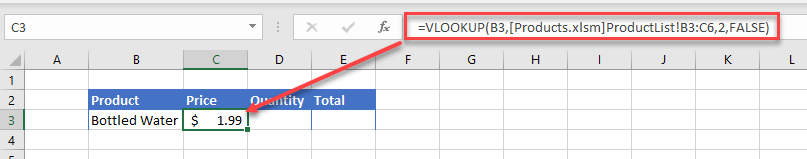To replicate this with VBA code, we would create the following macro:

``````Sub LookupPrice()
ActiveCell = Application.WorksheetFunction.VLookup(Range("B3"), Workbooks("Products.xlsm").Sheets("ProductList").Range("B2:C6"), 2, False)
End Sub``````

where the file Products.xlsm would need to be open in Excel for this lookup to work.

We could also use variables:

``````Sub LookupPrice()
Dim wb As Workbook
Dim ws As Worksheet
Dim rng As Range
Dim strProduct As String
Set wb = Workbooks("Products.xlsm")
Set ws = wb.Sheets("ProductList")
Set rng = ws.Range("B2:C6")
strProduct = Range("B3")
ActiveCell = Application.WorksheetFunction.VLookup(strProduct, rng, 2, False)
End Sub``````

The result of either of these examples would then be:If we wanted the actual formula to appear in the cell rather than the value, then our VBA code would then incorporate the workbook name as well as the sheet name or number.

For example:

``````Sub LookupPrice()
ActiveCell.FormulaR1C1 = "=VLOOKUP(RC[-1],[Products.xlsm]ProductList!R3C2:R6C3,2,FALSE)"
End Sub

``````

### XLOOKUP  from Another Workbook

Linking to another file using the XLOOKUP rather than the VLOOKUP follows the same lines.

To return the value to Excel:

``````Sub LookupPrice()
ActiveCell = Application.WorksheetFunction.XLookup(Range("B3"),  Workbooks("Products.xlsm").Sheets("ProductList").Range("B2:B6"), Workbooks("Products.xlsm").Sheets("ProductList").Range("C2:C6"))
End Sub``````

or with variables:

``````Sub LookupPrice()
Dim wb as Workbook
Dim ws As Worksheet
Dim strProduct As String
Dim rngProduct As Range
Dim rngPrice As Range
strProduct = Range("B3")
Set wb = Workbooks("Products.xlsm")
Set ws = wb.Sheets(ProductList)
Set rngProduct = ws.Range("B2:B6")
Set rngPrice = ws.Range("C2:C6")
ActiveCell = Application.WorksheetFunction.XLookup(strProduct, rngProduct, rngPrice)
End Sub``````

The result of either of these examples would then be:To return the formula to Excel rather than the value, we can amend our macro to include the workbook name as well as the sheet name or number.

``````Sub LookupPrice()
ActiveCell.Formula2R1C1 = "=XLOOKUP(RC[-1],[Products.xlsm]ProductList!RC[-1]:RC[-1],[Products.xlsm]ProductList!RC:RC)"
End Sub``````